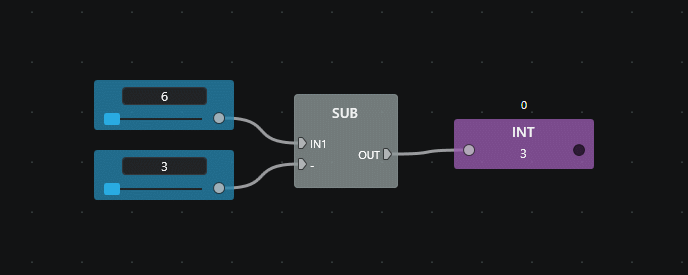# Arithmetic¶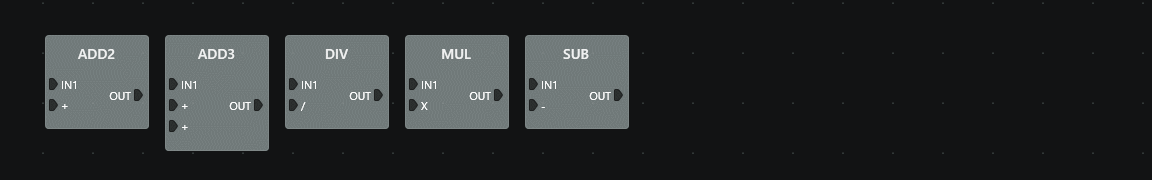Blocks that perform arithmetic operations. Note that you may use any numerical data type with these blocks.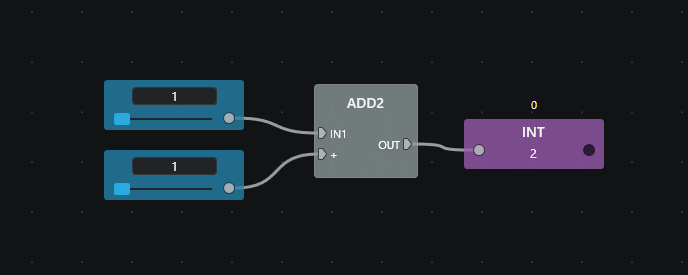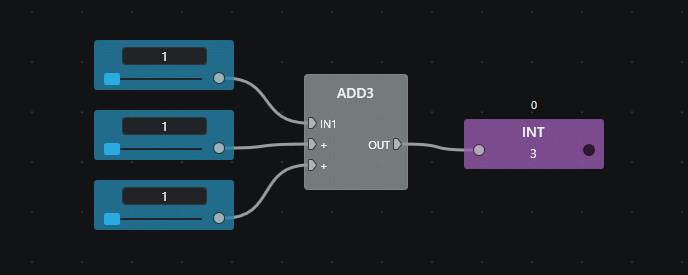## DIV¶

Divides two numbers.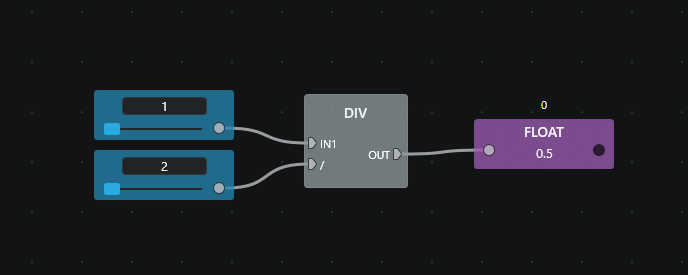## MUL¶

Multiplies two numbers.## SUB¶

Subtracts two numbers.